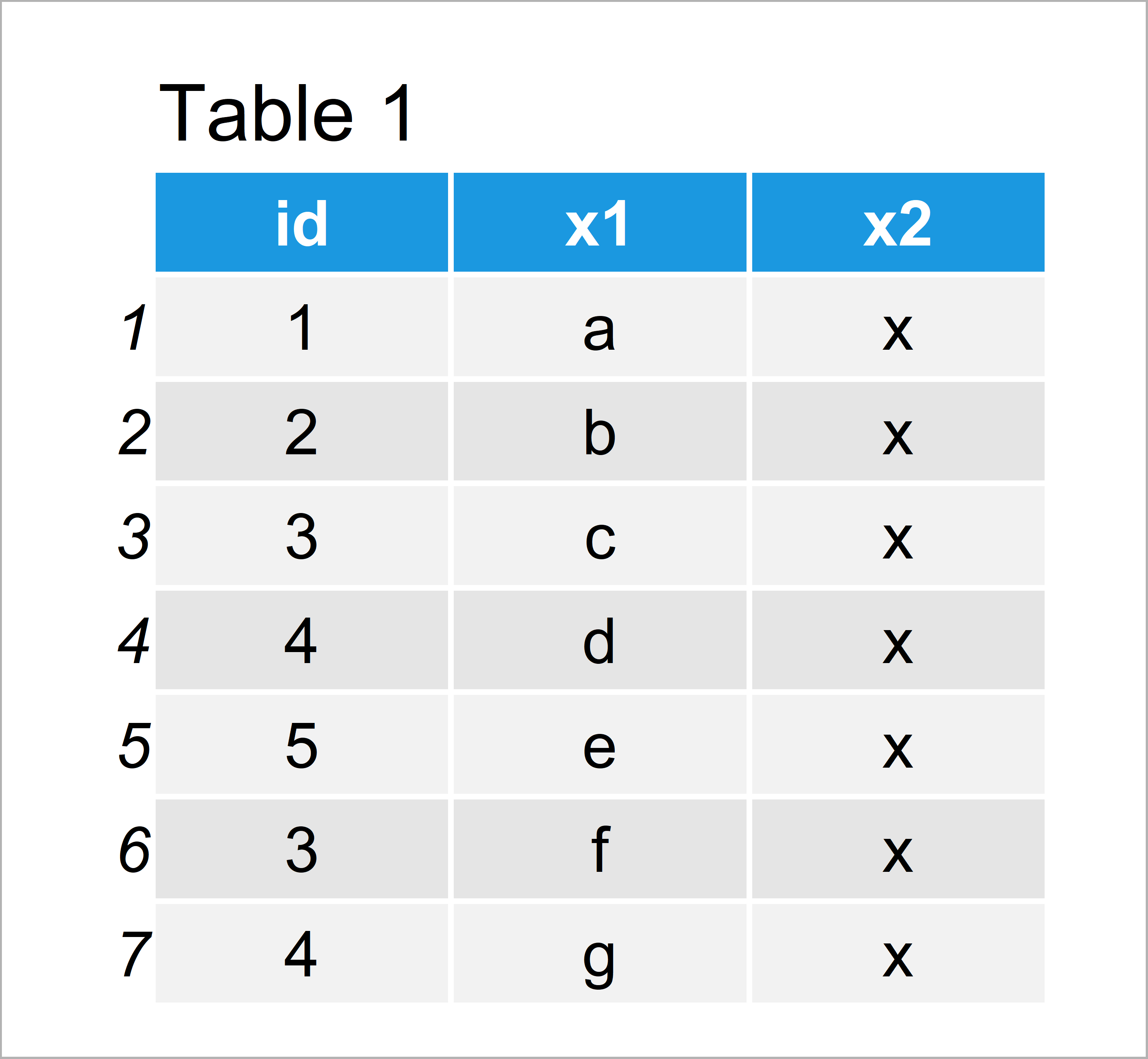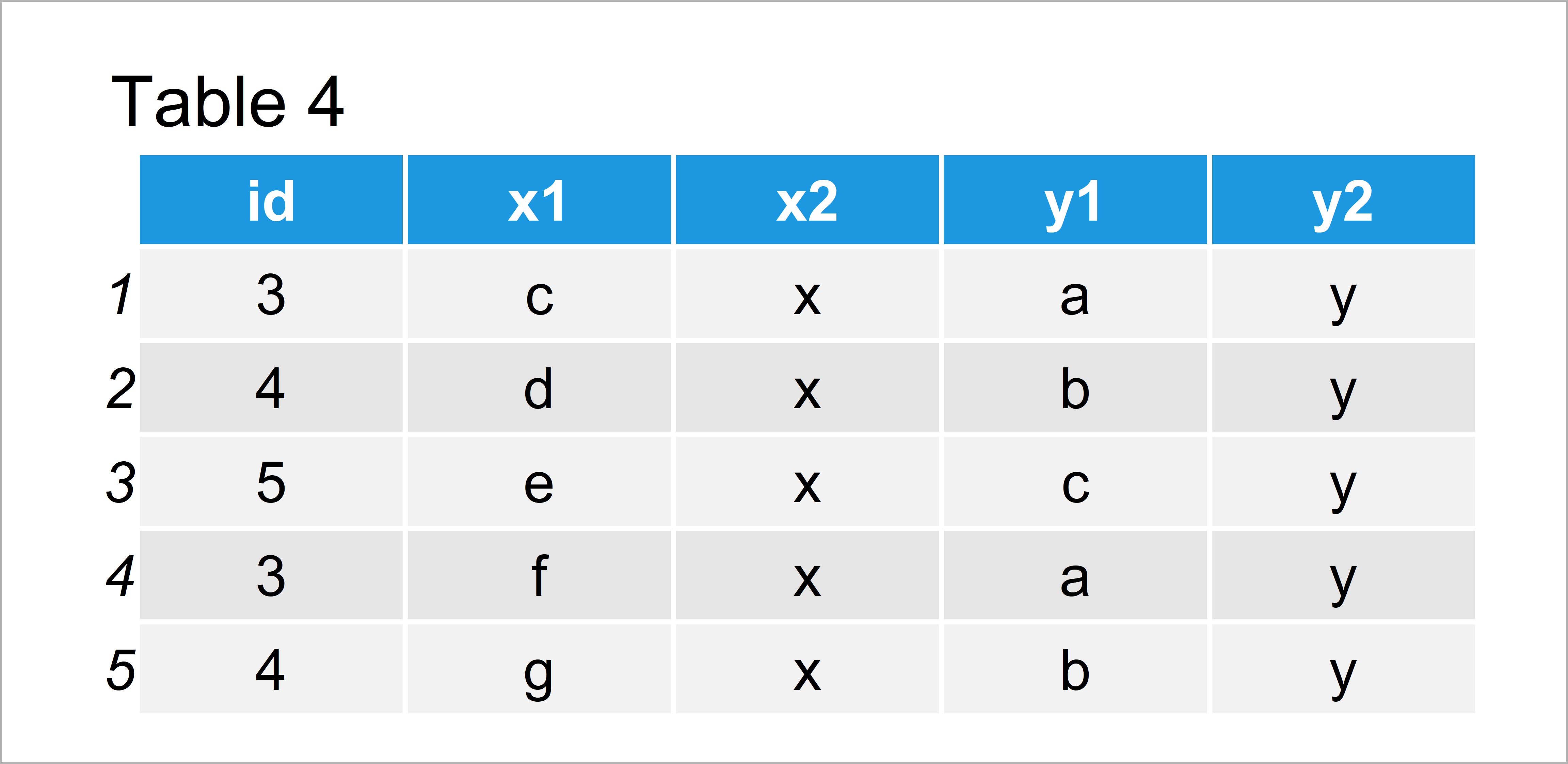# Keep Original Row Order when Merging Data (Example)

On this page, I’ll show how to retain the order of the rows of the first input data frame when merging data in R programming.

The tutorial will contain one example for the combination of data frames. To be more precise, the tutorial will consist of this information:

Let’s just jump right in:

## Example Data

The first step is to create some example data:

```data1 <- data.frame(id = c(1:5, 3, 4),    # Create first example data
x1 = letters[1:7],
x2 = "x")
data1                                     # Print first example data```Table 1 shows the structure of our first example data frame: It is composed of seven data points and three columns. Note that the id variable is not numerically ordered.

Let’s create another data frame in R:

```data2 <- data.frame(id = 3:6,             # Create second example data
y1 = letters[1:4],
y2 = "y")
data2                                     # Print second example data```As visualized in Table 2, we have created a second data frame containing different ids than the first data frame.

Using the basic installation of the R programming language, we could now merge these two data frames by column names using the merge() function:

```data_merge <- merge(data1, data2)         # Apply merge function
data_merge                                # Print merged data```The output of the previous R programming syntax is shown in Table 3: We have created a merged version of our two data frames.

However, as you can see the rows were automatically ordered according to the id column. While this might be convenient in some cases, it can definitely be annoying in other cases.

For that reason, I will show you how to keep the row order of a merged data set in the following example.

## Example: Keep Original Row Order when Merging Data Using join Functions of dplyr Package

In this section, I’ll show how to use the join functions of the dplyr package to keep the order of merged rows.

We first need to install and load the dplyr package to R.

```install.packages("dplyr")                 # Install dplyr package

Next, we can use one of the join functions (i.e. inner_join) to merge our data frames:

```data_join <- inner_join(data1, data2)     # Apply inner_join function
data_join                                 # Print joined data```Table 4 shows the output of the previous R programming code – We have merged our data frames and kept the row ordering of the first input data frame.

## Video & Further Resources

Would you like to know more about merging and joining data in R? Then you might want to have a look at the following video of my YouTube channel. In the video instruction, I’m explaining the content of the present page:

Besides that, you may read the related tutorials of this website:

In this R tutorial you have learned how to keep the row order after merging data frames. If you have any further questions, tell me about it in the comments section.

Subscribe to the Statistics Globe Newsletter